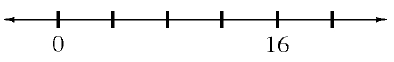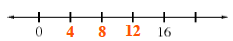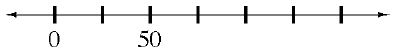### Home > CC1MN > Chapter 3 > Lesson 3.1.4 > Problem3-63

3-63.

Copy the incomplete axes and fill in the missing numbers to make the scaling consistent.

1.• Between $0$ and $16$, there are $4$ spaces.
Mark the axis to represent this.See the axis above.

1.• There are $2$ spaces which together represent $50$ units.
How many units does each space represent?